Courses

# Elasticity of Demand & Price Elasticity CA Foundation Notes | EduRev

## CA Foundation : Elasticity of Demand & Price Elasticity CA Foundation Notes | EduRev

The document Elasticity of Demand & Price Elasticity CA Foundation Notes | EduRev is a part of the CA Foundation Course Business Economics for CA Foundation.
All you need of CA Foundation at this link: CA Foundation

ELASTICITY OF DEMAND
Till now we were concerned with the direction of the changes in prices and quantities demanded. From the point of view of a business firm, it is more important to know the extent of the relationship or the degree of responsiveness of demand to changes in its determinants. Now we will try to measure these changes, or to say, we will try to answer the question “by how much does demand change due to a change in price”? Consider the following situations:
(1) As a result of a fall in the price of radio from ₹ 500 to ₹ 400, the quantity demanded increases from 100 radios to 150 radios.
(2) As a result of fall in the price of wheat from ₹ 20 per kilogram to ₹ 18 per kilogram, the quantity demanded increases from 500 kilograms to 520 kilograms.
(3) As a result of fall in the price of salt from ₹ 9 per kilogram to ₹ 7.50, the quantity demanded increases from 1000 kilogram to 1005 kilograms.
What do you notice? You notice that as a result of a fall in the price of radios, the quantity demanded of radios increases. Same is the case with wheat and salt. Thus, we can say that demand for radios, wheat and salt all respond to price changes. Then, what is the difference? The difference lies in the degree of response of demand which can be found out by comparing the percentage changes in prices and quantities demanded. Here lies the concept of elasticity.

Definition: Elasticity of demand is defined as the responsiveness of the quantity demanded of a good to changes in one of the variables on which demand depends. More precisely, elasticity of demand is the percentage change in quantity demanded divided by the percentage change in one of the variables on which demand depends.
These variables are price of the commodity, prices of the related commodities, income of the consumers and other factors on which demand depends. Thus, we have price elasticity, cross elasticity, income elasticity, advertisement elasticity and elasticity of substitution,. It is to be noted that when we talk of elasticity of demand, unless and until otherwise mentioned, we talk of price elasticity of demand. In other words, it is price elasticity of demand which is usually referred to as elasticity of demand.

Price Elasticity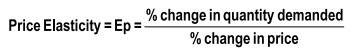or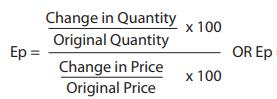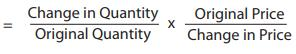In symbolic terms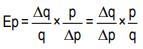Where
Ep stands for price elasticity
q stands for quantity
p stands for price
∆ stands for a very small change.

Strictly speaking, the value of price elasticity varies from minus infinity to approach zero from the negative sign, because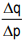has a negative sign. In other words, since price and quantity are inversely related (with a few exceptions) price elasticity is negative. But, for the sake of convenience, we ignore the negative sign and consider only the numerical value of the elasticity. Thus if a 1% change in price leads to 2% change in quantity demanded of good A and 4% change in quantity demanded of good B, then we get elasticity of A and B as 2 and 4 respectively, showing that demand for B is more elastic or responsive to price changes than that of A. Had we considered minus signs, we would have concluded that the demand for A is more elastic than that for B, which is not correct. Hence, by convention, we take the absolute value of price elasticity and draw conclusions.
A fews examples for price elasticity of demand case as follows:

Illustration 1:- The price of a commodity decreases from ₹ 6 to ₹ 4 and quantity demanded of the good increases from 10 units to 15 units. Find the coefficient of price elasticity.
Solution: Price elasticity = (-) ∆ q / ∆ p × p/q = 5/2 × 6/10 = (-) 1.5

Illustration 2:- A 5% fall in the price of a good leads to a 15% rise in its demand. Determine the elasticity and comment on its value.
Solution :-
Price elasticity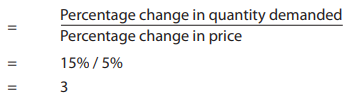Comment: The good in question has elastic demand.

Illustration 3:- The price of a good decreases from ₹ 100 to ₹ 60 per unit. If the price elasticity of demand for it is 1.5 and the original quantity demanded is 30 units, calculate the new quantity demanded.
Solution:-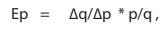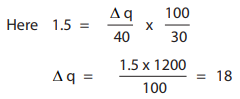Therefore new quantity demanded = 30+18 = 48 units .

Point elasticity: In point elasticity, we measure elasticity at a given point on a demand curve. The concept of point elasticity is used for measuring price elasticity where the change in price is infinitesimal. Point elasticity makes use of derivative rather than finite changes in price and quantity. It may be defined as: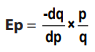whereis the derivative of quantity with respect to price at a point on the demand curve, and p and q are the price and quantity at that point. Point elasticity is, therefore, the product of price quantity ratio at a particular point on the demand curve and the reciprocal of the slope of the demand line.
It is to be noted that elasticity is different at different points on the same demand curve. Given a straight line demand curve tT, point elasticity at any point say R can be found by using the formula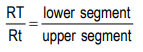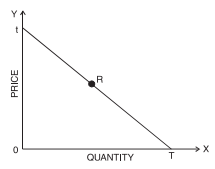Fig. 6 : Elasticity at a point on the demand curve
Using the above formula we can get elasticity at various points on the demand curve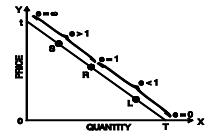Fig.: 6(a) : Elasticity at different
points on the demand curve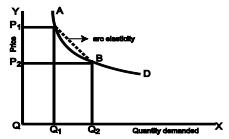Fig. 7 : Arc Elasticity

Thus, we see that as we move from T towards t, elasticity goes on increasing. At the mid-point it is equal to one, at point t it is infinity and at T it is zero.
Arc-elasticity: When the price change is somewhat larger or when price elasticity is to be found between two prices [or two points on the demand curve say, A and B in figure 7], the question arises which price and quantity should be taken as base. This is because elasticities found by using original price and quantity figures as base will be different from the one derived by using new price and quantity figures. Therefore, in order to avoid confusion, generally mid-point method is used i.e. the averages of the two prices and quantities are taken as (i.e. original and new) base. The arc elasticity can be found out by using the formula: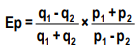where p1 , q1 are the original price and quantity and p2 , q2 are the new ones.

Thus, if we have to find elasticity of radios between:
p1 = ₹ 500    q1 = 100
p2 = ₹ 400   q2 = 150
We will use the formula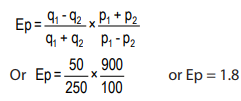Interpretation of the numerical values of elasticity of demand
The numerical value of elasticity of demand can assume any value between zero and infinity.
Elasticity is zero, if there is no change at all in the quantity demanded when price changes i.e. when the quantity demanded does not respond at all to a price change.
Elasticity is one, or unitary, if the percentage change in quantity demanded is equal to the percentage change in price.
Elasticity is greater than one when the percentage change in quantity demanded is greater than the percentage change in price. In such a case, demand is said to be elastic.
Elasticity is less than one when the percentage change in quantity demanded is less than the percentage change in price. In such a case, demand is said to be inelastic.
Elasticity is infinite, when a ‘small price reduction raises the demand from zero to infinity. Under such a case, consumers will buy all that they can obtain of the commodity at some price. If there is a slight increase in price, they would not buy anything from the particular seller. This type of demand curve is found in a perfectly competitive market.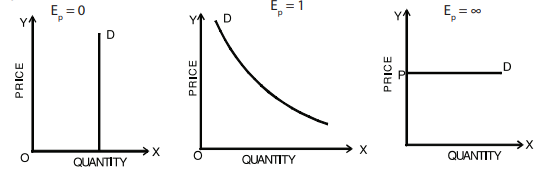Fig. 8(a) : Demand curve of zero, unitary and infinite elasticity

Elasticity is greater than one (Ep > 1) Elasticity is less than one (Ep < 1)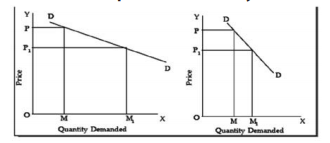Fig. 8(b) : Demand curves of greater than one and less than one elasticities

Table 4 : Elasticity measures, meaning and nomenclature

 Numerical measure of elasticity Verbal description Terminology Zero Quantity demanded does not change as price changes Perfectly (or completely) inelastic Greater than zero, but less than one Quantity demanded changes by a smaller percentage than does price Inelastic One Quantity demanded changes by exactly the same percentage as does price Unit elasticity Greater than one, but less than infinity Quantity demanded changes by a larger percentage than does price Elastic Infinity Purchasers are prepared to buy all they can obtain at some price and none at all at an even slightly higher price Perfectly (or infinitely) elastic

Now that we are able to classify goods according to their price elasticity, let us see whether the goods which we considered in our example on page 2.37, are price elastic or inelastic

 Sr. No. Name of the commodity Calculation of Elasticity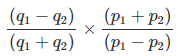Nature of Elasticity 1 Radios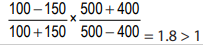Elastic 2 Wheat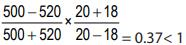Inelastic 3 Common Salt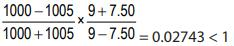Inelastic

What do we note in the above hypothetical example? We note that the demand for radios is quite elastic, while demand for wheat is quite inelastic and the demand for salt is almost the same even after a reduction in price.
Generally, in real world situations also, we find that demand for goods like radios, TVs, refrigerators, fans, etc. is elastic; demand for goods like wheat and rice is inelastic; and demand for salt is highly inelastic or perfectly inelastic. Why do we find such a difference in the behaviour of consumers in respect of different commodities? We shall explain later at length those factors which are responsible for the differences in elasticity of demand for various goods. First, we will consider another method of calculating price-elasticity which is called total outlay method.
Total Outlay Method of Calculating Price Elasticity: The price elasticity of demand for a commodity and the total expenditure or outlay made on it are greatly related to each other. As the total expenditure (price of the commodity multiplied by the quantity of that commodity purchased) made on a commodity is the total revenue received by the seller (price of the commodity multiplied by quantity of that commodity sold of that commodity), we can say that the price elasticity and total revenue received are closely related to each other. By analysing the changes in total expenditure (or revenue), we can know the price elasticity of demand for the good. However, it should be noted that by this method we can only say whether the demand for a good is elastic or inelastic; we cannot find out the exact coeficient of price elasticity.

Table 5: The Relationship between Price elasticity and Total Revenue (TR)

Demand

 Elastic Unitary Elastic Inelastic Price Increase TR Decrease TR Remain Same TR Increase Price Decrease TR Increase TR REmain Same TR Decrease

Determinants of Price Elasticity of Demand: In the above section we have explained what is price elasticity and how it is measured. Now an important question is: what are the factors which determine whether the demand for a good is elastic or inelastic? We will consider the following important determinants of price elasticity
(1) Availability of substitutes: One of the most important determinants of elasticity is the degree of availability of close substitutes. Some commodities like butter, cabbage, Maruti Car, Coca Cola, etc. have close substitutes. There are margarine, other green vegetables, Santro or other cars, Pepsi or any other cold drink respectively. A change in the price of these commodities, the prices of the substitutes remaining constant, can be expected to cause quite substantial substitution – a fall in price leading consumers to buy more of the commodity in question and a rise in price leading consumers to buy more of the substitutes. Commodities such as salt, housing, and all vegetables taken together, have few, if any, satisfactory substitutes and a rise in their prices may cause a smaller fall in their quantity demanded. Thus, we can say that goods which typically have close or perfect substitutes have highly elastic demand curves. Moreover, wider the range of substitutes available, the greater will be the elasticity. For example, toilet soaps, toothpastes etc have wide variety of brands and each brand is a close substitute for the other.
It should be noted that while as a group, a good or service may have inelastic demand, but when we consider its various brands, we say that a particular brand has elastic demand. Thus, while the demand for a generic good like petrol is inelastic, the demand for Indian Oil’s petrol is elastic. Similarly, while there are no general substitutes for health care, there are substitutes for one doctor or hospital. Likewise, the demand for common salt and sugar is inelastic because good substitutes are not available for these.
(2) Position of a commodity in a consumer’s budget: The greater the proportion of income spent on a commodity; generally the greater will be its elasticity of demand and vice- versa. The demand for goods like common salt, matches, buttons, etc. tend to be highly inelastic because a household spends only a fraction of their income on each of them. On the other hand, demand for goods like clothing, tends to be elastic since households generally spend a good part of their income on clothing.
(3) Nature of the need that a commodity satisfies: In general, luxury goods are price elastic while necessities are price inelastic. Thus, while the demand for television is relatively elastic, the demand for food and housing, in general, is inelastic. If it is possible to postpone the consumption of a particular good, such good will have elastic demand. Consumption of necessary goods cannot be postponed and therefore, their demand is inelastic.
(4) Number of uses to which a commodity can be put: The more the possible uses of a commodity, the greater will be its price elasticity and vice versa. When the price of a commodity which has multiple uses decreases, people tend to extend their consumption to its other uses. To illustrate, milk has several uses. If its price falls, it can be used for a variety of purposes like preparation of curd, cream, ghee and sweets. But, if its price increases, its use will be restricted only to essential purposes like feeding the children and sick persons.
(5) Time period: The longer the time-period one has, the more completely one can adjust. A simple example of the effect can be seen in motoring habits. In response to a higher petrol price, one can, in the short run, make fewer trips by car. In the longer run, not only can one make fewer trips, but he can purchase a car with a smaller engine capacity when the time comes for replacing the existing one. Hence one’s demand for petrol falls by more when one has made long term adjustment to higher prices.
(6) Consumer habits: If a consumer is a habitual consumer of a commodity, no matter how much its price change, the demand for the commodity will be inelastic.
(7) Tied demand: The demand for those goods which are tied to others is normally inelastic as against those whose demand is of autonomous nature. For example printers and ink cartridges.
(8) Price range: Goods which are in very high price range or in very low price range have inelastic demand, but those in the middle range have elastic demand.

Knowledge of the price elasticity of demand and the factors that may change it is of key importance to business managers because it helps them recognise the effect of a price change on their total sales and revenues. Firms aim to maximise their profits and their pricing strategy is highly decisive in attaining their goals. Price elasticity of demand for the goods they sell helps them in arriving at an optimal pricing strategy. If the demand for a firm’s product is relatively elastic, the managers need to recognize that lowering the price would expand the volume of sales, and result in an increase in total revenue. On the other hand, if demand were relatively inelastic, the firm may safely increase the price and thereby increase its total revenue as they know that the fall in sales would be less than proportionate. On the contrary, if demand is elastic, a price increase will lead to a decline in total revenue as fall in sales would be more than proportionate. Knowledge of price elasticity of demand is important for governments while determining the prices of goods and services provided by them, such as, transport and telecommunication. Further, it also helps the governments to understand the nature of responsiveness of demand to the increase in prices on account of additional taxes and the implications of such responses on the tax revenues. Elasticity of demand explains why Governments are inclined to raise the indirect taxes on those goods that have a relatively inelastic demand, like alcohol and tobacco products.

Offer running on EduRev: Apply code STAYHOME200 to get INR 200 off on our premium plan EduRev Infinity!

## Business Economics for CA Foundation

61 videos|111 docs|67 tests

,

,

,

,

,

,

,

,

,

,

,

,

,

,

,

,

,

,

,

,

,

;